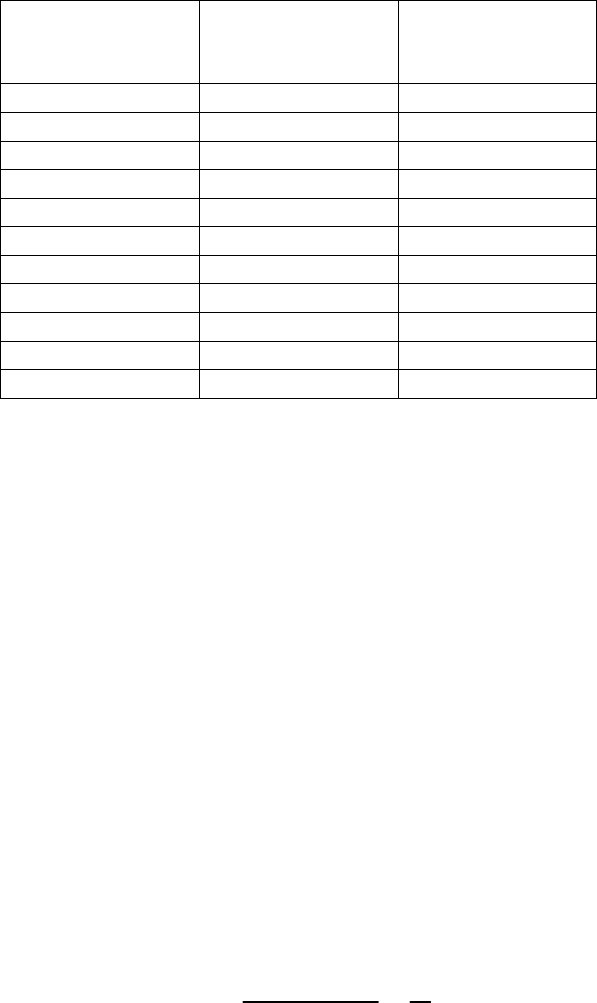Class Notes (1,100,000)
CA (620,000)
Concordia (5,000)
COMM (600)
Lecture 4

# COMM 220 Lecture Notes - Lecture 4: Prentice Hall, Production Function, Technological Change

Department
Commerce
Course Code
COMM 220
Professor
Loretta Hung
Lecture
4

This preview shows pages 1-3. to view the full 14 pages of the document.
Source: Pindyck and Rubinfeld (2009), Microeconomics, 7
th
Ed., Pearson Prentice Hall, Chapter 6.
1
CHAPTER 6 – PRODUCTION
Key Concepts and Topics
The Technology of Production
Production with One Variable Input (Labor)
Isoquants
Production with Two Variable Inputs
Returns to Scale
Introduction
Theory of the firm
Explains how a firm makes cost-minimizing production decisions and how
its costs vary with output. Costs of production change with changes in both
input prices and level of output
Production Decisions of a Firm
Production decisions of a firm can be broken down into three steps:
1. Production Technology
2. Cost Constraints
3. Input Choices
Production Technology
Describe how inputs can be transformed into outputs
Inputs: land, labor, capital and raw materials
Outputs: cars, desks, books, etc.
Firms can produce different amounts of outputs using different combinations
of inputs
Cost Constraints
Firms must consider prices of labor, capital and other inputs
Firms want to minimize total production costs partly determined by input
prices
Firms must be concerned about costs of production
Input Choices
Given input prices and production technology, the firm must choose how
much of each input to use in producing output

Only pages 1-3 are available for preview. Some parts have been intentionally blurred.

Source: Pindyck and Rubinfeld (2009), Microeconomics, 7
th
Ed., Pearson Prentice Hall, Chapter 6.
2
Given prices of different inputs, the firm may choose different combinations
of inputs to minimize costs
If labor is cheap, may choose to produce with more labor and less capital
If a firm’s costs of production are at its minimization, we can study
How total costs of production varies with output
How does the firm choose the quantity to maximize its profits
We can represent the firm’s production technology in the form of a production
function
The Technology of Production
Production Function
Describes the maximum output (q) that a firm can produce for every
specified combination of inputs at a given state of technology
For simplicity, we will consider only labor (L) and capital (K)
Shows what is technically feasible when the firm operates efficiently
The production function for two inputs: q = F(K,L)
Output (q) is a function of capital (K) and labor (L)
Both inputs and output are flows
The production function is true for a given technology
If technology increases, more output can be produced for a given level of
inputs
Short Run versus Long Run
It takes time for a firm to adjust production from one set of inputs to another
Firms must consider not only what inputs can be varied but over what period
of time that can occur
We must distinguish between long run and short run
Short run and long run are not time specific
Short Run
Period of time in which at least one of the inputs is fixed
For example, when K is fixed, firms vary the intensity with which they
utilize a given plant and machinery
Long-run
Amount of time needed to make all production inputs variable

Only pages 1-3 are available for preview. Some parts have been intentionally blurred.Source: Pindyck and Rubinfeld (2009), Microeconomics, 7
th
Ed., Pearson Prentice Hall, Chapter 6.
3
Production with One Variable Input (Labor)
Assume K is fixed and L is variable (i.e., a short run analysis)
Output can only be increased by increasing L
Amount of
Labor
(L)
Amount of
Capital
(K)
Total Output
(q)
0 10 0
1 10 10
2 10 30
3 10 60
4 10 80
5 10 95
6 10 108
7 10 112
8 10 112
9 10 108
10 10 100
Observations: How output changes as the amount of labor is changed?
When labor is zero, output is zero
With additional workers, output increases up to 8 units of labor
Beyond 8 units of labor, output declines
Increasing labor can make better use of existing capital initially
After a point, more labor is not useful and can be counterproductive
Firms make decisions based on the benefits and costs of production
Sometimes useful to look at benefits and costs on an incremental basis
How much more can be produced with an additional unit of an input
Sometimes useful to make comparison on an average basis
Average Product (AP)
Output per unit of a particular input
Average Product of Labor (AP
L
)
Measures the productivity of a firm’s labor in terms of how much, on
average, each worker can produce
L
q
LaborInput
Output
AP
L
==